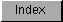```
The HorizPot and VertPot variables contain the actual proportional values
entered into or displayed by the gadget. The word pot is short for
potentiometer, which is an electrical analog device used to adjust a value
within a continuous range.

The proportional gadget pots allow the program to set the current position
of the knob within the container, or to read the knob's current location.

The pot variable is a 16-bit, unsigned variable that contains a value
ranging from zero to 0xFFFF. For clarity, the constant MAXPOT is
available, which is equivalent to 0xFFFF. A similar constant MAXBODY is
available for the body variables. As the pot variables are only 16 bits,
the resolution of the proportional gadgets has a maximum of 65,536
positions (zero to 65,535).

The values represented in the pot variables are usually translated or
converted to a range of numbers more useful to the application.  For
instance, if a slider covered the range one to three, pot values of zero
to 16,383 would represent one, values of 16,384 to 49,151 would represent
two and values of 49,152 to 65,535 would represent three.  The method of
deriving these numbers is fairly complex, refer to the sample code below